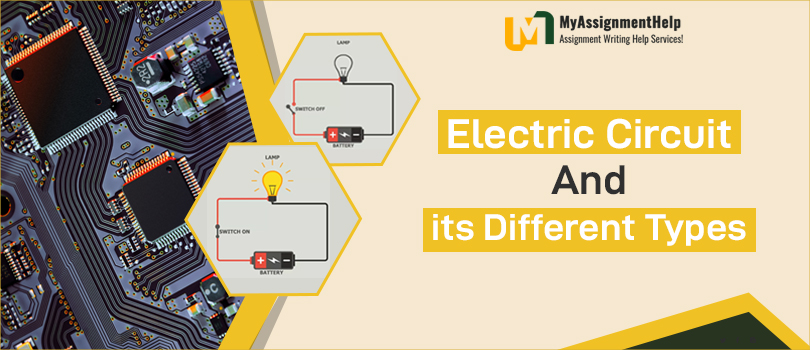A circuit is a set of active and passive electronic components including resistors, capacitors, inductors, diodes, transistors, etc. that combine to form a circuit. During a closed-loop circuit, electric current travels from the source (such as battery) to the load (similar to a lamp) and then returns to the source.An electric network is a set of electronic elements and components which are connected in various ways (simple or complex configurations). A network theorem solves complex networks by applying the same term commonly used for electric circuits.  let’s get more deeper about electricity terms from Electric Circuits Assignment Help

## Complex Networks of Electric Circuit

Components and elements of a complex network are resistors, capacitors, inductors, as well as AC and DC voltage sources and current sources. Neither Ohm’s Law nor Kirchhoff’s Law can solve these types of networks easily. As a result, they have more equations. There are particular techniques that can be applied to solve and analyze a complex network such as network theorems, such as the Norton’s theorem, Thevenin’s theorem, superposition theorem, star-delta transformation, supernode and supermesh circuit analysis.

Linear Circuits: Circuits with linear characteristics (resistance, inductance, capacitance, frequency, waveform, etc) are constant in terms of the circuit parameters. A linear circuit is one whose parameters do not change when current and voltage are increased or decreased. There are many assignment writing service available on the internet to get your assignments done in exact timelimit.

Nonlinear Circuits: An electric circuit whose parameters are changed according to voltage and current is referred to as a nonlinear circuit. In other words, an electric circuit with parameters such as resistance, inductance, capacitance, waveform, and frequency that are not constant is referred to as a non-linear circuit.

Unilateral Circuits: As the voltage or current direction changes in unilateral circuits, so does the property of the circuit. An unilateral circuit is one in which current flows only one way. In a unilateral circuit, a diode or rectifier performs rectification in only one direction. You can buy assignment help to get your work done and still score high grades.

Bi-lateral Circuits: If the current or voltage supply direction changes in bilateral circuits, the property of the circuit remains the same. The current can then flow in both directions in a bilateral circuit. A transmission line is a bilateral circuit since its properties remain constant no matter from which end the supply voltage originates.

### Circuits and Networks Terms Related to Electricity

Node: When two or more elements of a circuit (such as resistors, capacitors, conductors, etc) meet, it’s called a node

Branch: Circuits that have parts or sections between two junctions are called branches. There can be more than one element with two terminals in a branch.

Loop: Among closed paths in a circuit, a loop occurs when more than two meshes are possible. The loop may contain one or many meshes, but the mesh itself does not contain a loop.

Mesh: An area that contains only a single closed loop within it and a single path is referred to as a mesh.

### Know BookMyEssay a Bit More

A student can use BookMyEssay for writing assignments, essays, and many other kinds of academic writing. Professionals and experts from this platform are the ones who are specialized in their fields. BookMyEssay has much more to offer their students than just writing services because modern problems require out-of-the-box solutions, which is why BookMyEssay is the best choice for students seeking assistance.